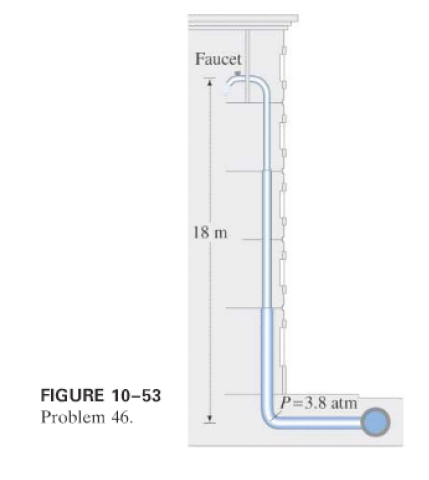×
Get Full Access to Physics: Principles With Applications - 6 Edition - Chapter 10 - Problem 46p
Get Full Access to Physics: Principles With Applications - 6 Edition - Chapter 10 - Problem 46p

×

# Water at a gauge pressure of 3.8 atm at street level flowsISBN: 9780130606204 3

## Solution for problem 46P Chapter 10

Physics: Principles with Applications | 6th Edition

• Textbook Solutions
• 2901 Step-by-step solutions solved by professors and subject experts
• Get 24/7 help from StudySoup virtual teaching assistantsPhysics: Principles with Applications | 6th Edition

4 5 1 263 Reviews
17
2
Problem 46P

(II) Water at a gauge pressure ofatm at street level flows into an office building at a speed ofthrough a pipein diameter. The pipe tapers down toin diameter by the top floor,above (Fig.), where the faucet has been left open. Calculate the flow velocity and the gauge pressure insuch a pipe on the top floor. Assume no branch pipes and ignore viscosity.Step-by-Step Solution:
Step 1 of 3

ANSWER:A)Mass of the car is 710 kg.Speed of the car is 23 m/s.Drag force is, F drag= 500 N .As there are various types of drag forces, the way of finding the power is same. So,the power required in the presence of drag force is, P = F × velocity ---------------------(1) d P = 500 × 23 = 11500 watts.This is the power required to move on the horizontal surface of ground.B) 0While riding the inclined hill which make s an angle of 3 with the horizontal, the things willbe changed a little.Here it has to overcome the frictional force with the drag force as well.As the normal reaction will cancel the vertical component of the force, only the mg Sin term will contribute for the frictional force.So the force the car has to overcome is, 0 F = mg sin + F D = (710 × 9.8 × sin 2 ) + 500 = 242.83 + 500 = 742.83N.\nAs we know the power is, P = F × velocity = 742.83 × 23 = 17085watts.So, the required power is 17085 watts.

Step 2 of 3

Step 3 of 3

##### ISBN: 9780130606204

Physics: Principles with Applications was written by and is associated to the ISBN: 9780130606204. Since the solution to 46P from 10 chapter was answered, more than 448 students have viewed the full step-by-step answer. This textbook survival guide was created for the textbook: Physics: Principles with Applications, edition: 6. The answer to “?(II) Water at a gauge pressure of atm at street level flows into an office building at a speed of through a pipe in diameter. The pipe tapers down to in diameter by the top floor, above (Fig. ), where the faucet has been left open. Calculate the flow velocity and the gauge pressure in such a pipe on the top floor. Assume no branch pipes and ignore viscosity.” is broken down into a number of easy to follow steps, and 69 words. The full step-by-step solution to problem: 46P from chapter: 10 was answered by , our top Physics solution expert on 03/03/17, 03:53PM. This full solution covers the following key subjects: pipe, diameter, pressure, top, Gauge. This expansive textbook survival guide covers 35 chapters, and 3914 solutions.

## Discover and learn what students are asking

Calculus: Early Transcendental Functions : Conics, Parametric Equations, and Polar Coordinates
?In Exercises 1–6, match the equation with the correct graph. [The graphs are labeled (a), (b), (c), (d), (e), and (f).]

Statistics: Informed Decisions Using Data : Inference about the Difference between Two Medians: Dependent Samples
?In Problems 3–10, use the Wilcoxon matched-pairs signedranks test to test the given hypotheses at the a = 0.05 level of significance. The dependent sa

Unlock Textbook Solution